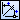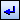# Creating Ordinate Angular Dimension

Create an ordinate angular dimension by locating dimension lines continuously on the same circle using the start point as the base point.

1. Point [Ordinate Dimension] on [Shape] menu, and click [Ordinate Angular Dimension].
Then you will be able to create an ordinate angular dimension.
2.  Supplemental ▪ You can enter into the ordinate angular dimension creating status by clickingon [Shape] toolbar.
3. Click the center point of an ordinate angular dimension on the drawing.
4. Click the start point of an ordinate angular dimension on the drawing.
5. Click the end point of the first dimension line on the drawing.
The angle between the start and end points will be automatically set as a dimension value.
6. Specify whether to locate the dimension value on the auxiliary line on the end point side, or on the end point side of the dimension, in [Dimension Value Place] box on Command Window.
7. Click a position where a dimension line is to be located on the drawing.
A dimension line will be located at the clicked position.
8. Click end points of the second and subsequent dimension lines on the drawing in order.
Each time you click the end point, dimension lines using the start point as the base will be created continuously on the same circle, for example the dimension line connecting the secondly-specified end point and start point, that connecting the third-specified end point and start point, and so on.
9. Press Enter key on the keyboard, or clickon the toolbar on Command Window.
Specifying end points will be complete, and an ordinate angular dimension will be created.
 Supplemental ▪ You can also specify the distance from the start/end point to the first dimension line by switching [Locate Point] box to [Distance from Base Point] box. In such a case, specify a point locating the dimension line in [Direction Point] box. ▪ You can specify the center point, start point, end point, locate point, direction point and end point of after second dimension by inputting X and Y coordinates in [Center Point], [Start Point], [End Point], [Locate Point], [Direction Point] and [End Point for Second and Subsequent Dimensions] boxes on Command Window.